# Aswath Damodaran VALUATION QUIZ 1 REVIEW Aswath Damodaran

• Slides: 20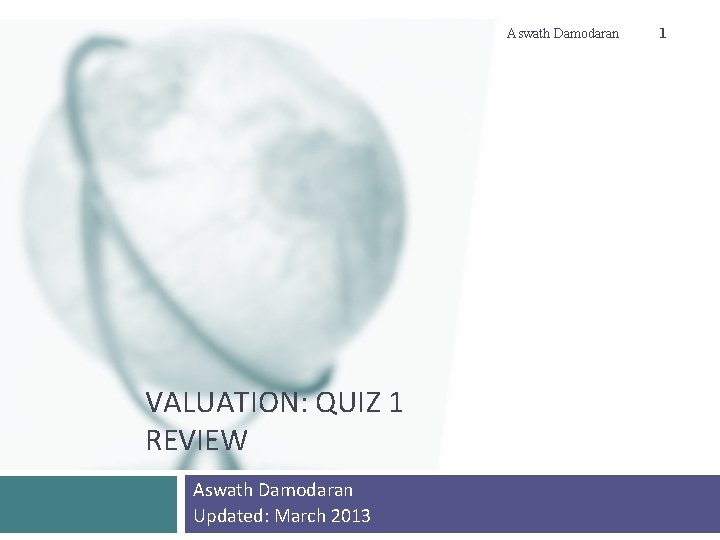Aswath Damodaran VALUATION: QUIZ 1 REVIEW Aswath Damodaran Updated: March 2013 1Key topics covered 2 Valuation Approaches Basics of DCF valuation The essence of intrinsic value Equity versus Firm valuation DCF consistency Discount Rates What are three approaches to valuation? From a big picture perspective, what are the key differences between the different valuation approaches? What are the broad factors that determine which approach to use? Risk free rates: How to estimate and why they vary across currencies Equity Risk Premium: Historical vs Implied, Mature & Country Risk Premiums Company risk exposure to country risk Company risk exposure to macro risk (beta and other relative risk measures) Cost of debt and capital Cash flows Updating and normalizing earnings Cleaning up earnings for accounting inconsistencies (leases & R&D) Tax rates and taxes Capital expenditures, working capital and reinvestment From cash flow to the firm to cash flow to equity Aswath Damodaran 2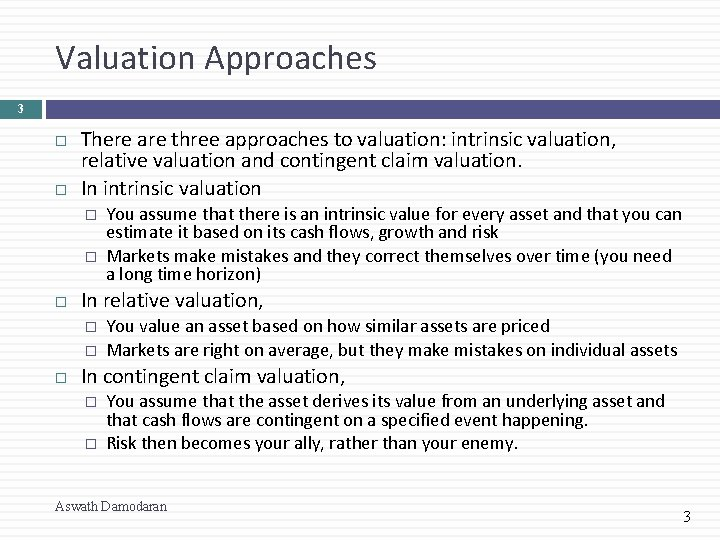Valuation Approaches 3 There are three approaches to valuation: intrinsic valuation, relative valuation and contingent claim valuation. In intrinsic valuation � � In relative valuation, � � You assume that there is an intrinsic value for every asset and that you can estimate it based on its cash flows, growth and risk Markets make mistakes and they correct themselves over time (you need a long time horizon) You value an asset based on how similar assets are priced Markets are right on average, but they make mistakes on individual assets In contingent claim valuation, � � You assume that the asset derives its value from an underlying asset and that cash flows are contingent on a specified event happening. Risk then becomes your ally, rather than your enemy. Aswath Damodaran 3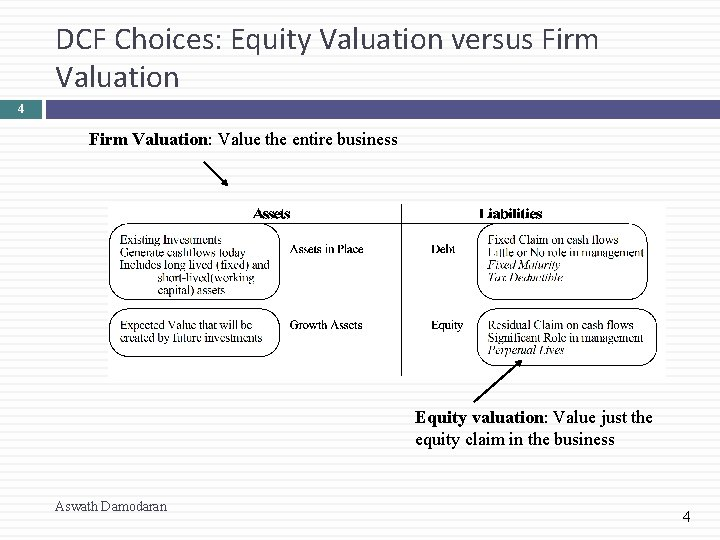DCF Choices: Equity Valuation versus Firm Valuation 4 Firm Valuation: Value the entire business Equity valuation: Value just the equity claim in the business Aswath Damodaran 4Quiz problem: Consistency 5 You have been asked to review the valuation of Santiago Cement, a small Peruvian cement company, by an M&A analyst, for acquisition by a US cement company. The analyst has estimated a value of 1 billion Peruvian Sol for the equity, based upon the expectation that the firm will generate 50 million Peruvian sol in cash flows (to equity) next year, growing at 5% (in sol) a year forever; mistakenly, he used the US company’s dollar cost of equity in the valuation. To correct the valuation, you have been provided with the following information: The US T. Bond rate is 3% and Peruvian US\$ denominated bond rate is 5%; Peruvian equities are 1. 5 times more volatile than the Peruvian \$ bond. The expected inflation rate in Peruvian sol is 6% and the expected inflation rate in US dollars is 2%. The typical Peruvian company generates 80% of its revenues in Peru, but Santiago Cement generates all of its revenues in Peru. Estimate the correct value of equity in Santiago Cement. Aswath Damodaran 5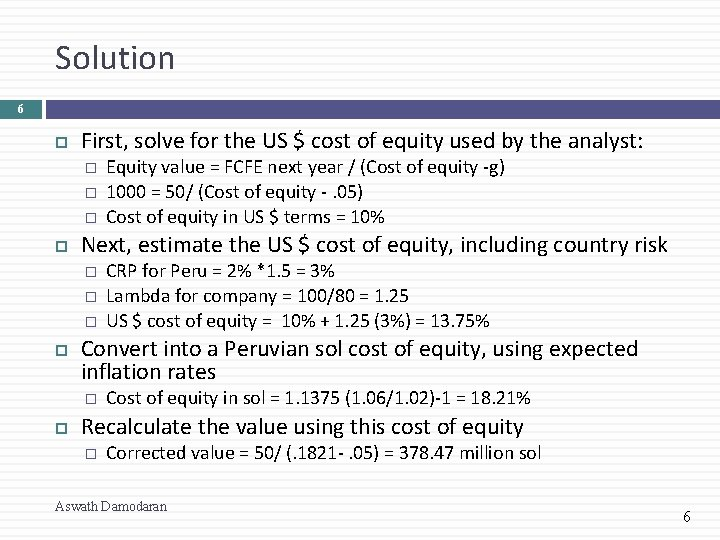Solution 6 First, solve for the US \$ cost of equity used by the analyst: � � � Next, estimate the US \$ cost of equity, including country risk � � � CRP for Peru = 2% *1. 5 = 3% Lambda for company = 100/80 = 1. 25 US \$ cost of equity = 10% + 1. 25 (3%) = 13. 75% Convert into a Peruvian sol cost of equity, using expected inflation rates � Equity value = FCFE next year / (Cost of equity -g) 1000 = 50/ (Cost of equity -. 05) Cost of equity in US \$ terms = 10% Cost of equity in sol = 1. 1375 (1. 06/1. 02)-1 = 18. 21% Recalculate the value using this cost of equity � Corrected value = 50/ (. 1821 -. 05) = 378. 47 million sol Aswath Damodaran 6Inputs to cost of equity: The Macro numbers 7 Riskfree rate: For an investment to be riskfree, then, it has to have � � No default risk No reinvestment risk Equity Risk Premium: This is the premium that you charge for investing in equities as a class. While it is often estimated looking at history, these historical premiums tend to have high standard errors. An alternative is to estimate an implied premium by looking at how stocks are priced today. � Country Risk Premium: To the extent that you are investing in countries which are not mature, you should charge an extra risk premium, which can be estimated by � � � The default spread for the country The default spread scaled up for the additional risk of equities Aswath Damodaran 7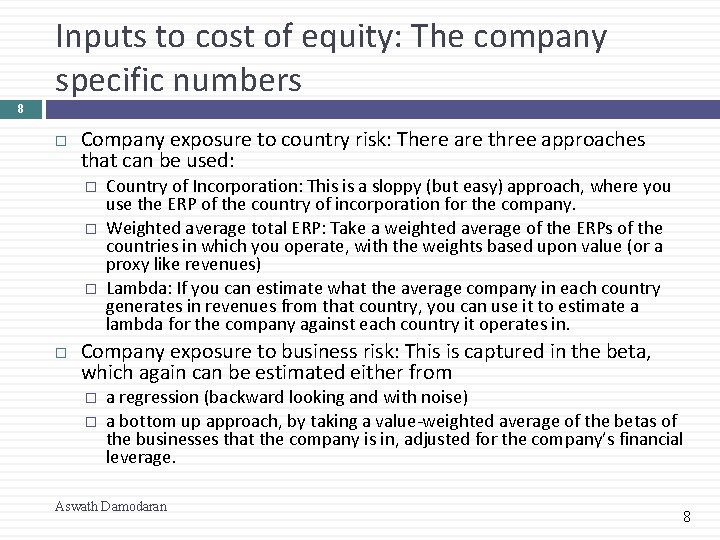Inputs to cost of equity: The company specific numbers 8 Company exposure to country risk: There are three approaches that can be used: � � � Country of Incorporation: This is a sloppy (but easy) approach, where you use the ERP of the country of incorporation for the company. Weighted average total ERP: Take a weighted average of the ERPs of the countries in which you operate, with the weights based upon value (or a proxy like revenues) Lambda: If you can estimate what the average company in each country generates in revenues from that country, you can use it to estimate a lambda for the company against each country it operates in. Company exposure to business risk: This is captured in the beta, which again can be estimated either from � � a regression (backward looking and with noise) a bottom up approach, by taking a value-weighted average of the betas of the businesses that the company is in, adjusted for the company’s financial leverage. Aswath Damodaran 8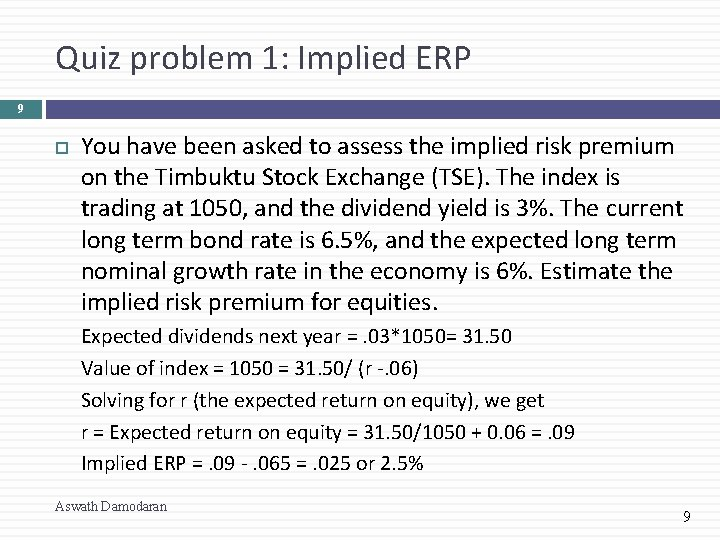Quiz problem 1: Implied ERP 9 You have been asked to assess the implied risk premium on the Timbuktu Stock Exchange (TSE). The index is trading at 1050, and the dividend yield is 3%. The current long term bond rate is 6. 5%, and the expected long term nominal growth rate in the economy is 6%. Estimate the implied risk premium for equities. Expected dividends next year =. 03*1050= 31. 50 Value of index = 1050 = 31. 50/ (r -. 06) Solving for r (the expected return on equity), we get r = Expected return on equity = 31. 50/1050 + 0. 06 =. 09 Implied ERP =. 09 -. 065 =. 025 or 2. 5% Aswath Damodaran 9Quiz problem 2: Risk free rates & Equity Risk Premiums 10 You are reviewing the cost of equity computation that an analyst has made for Luo Tang, a Vietnamese company. The analyst has estimated a cost of equity of 18% for the company in Vietnamese Dong (VND). In making this estimate, the analyst used the following information: � � The ten-year Vietnamese government bond rate, in VND, is 9%, and was used by the analyst as the riskfree rate. However Vietnam has a local currency rating of Baa 3 and the default spread for Baa 3 rated bonds is 3%. The analyst used a total equity risk premium of 7. 5% for Vietnam (obtained by adding 3% to the US risk premium of 4. 5%) and a beta of 1. 2 for the company. Here is the rest of the information � � The volatility in the Vietnamese equity index is twice the volatility in the Vietnamese government bond. Only 30% of Luo Tang’s revenues come from Vietnam and that the rest come from mature markets, while the average Vietnamese company gets 90% of its revenues from Vietnam. Aswath Damodaran 10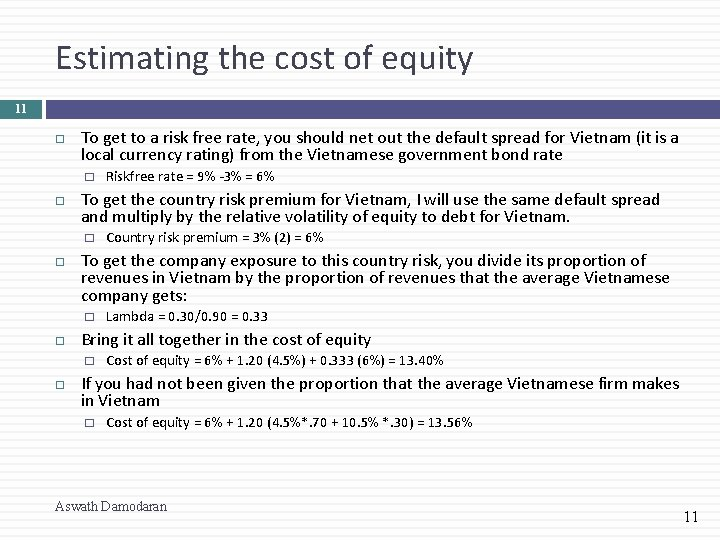Estimating the cost of equity 11 To get to a risk free rate, you should net out the default spread for Vietnam (it is a local currency rating) from the Vietnamese government bond rate � To get the country risk premium for Vietnam, I will use the same default spread and multiply by the relative volatility of equity to debt for Vietnam. � Lambda = 0. 30/0. 90 = 0. 33 Bring it all together in the cost of equity � Country risk premium = 3% (2) = 6% To get the company exposure to this country risk, you divide its proportion of revenues in Vietnam by the proportion of revenues that the average Vietnamese company gets: � Riskfree rate = 9% -3% = 6% Cost of equity = 6% + 1. 20 (4. 5%) + 0. 333 (6%) = 13. 40% If you had not been given the proportion that the average Vietnamese firm makes in Vietnam � Cost of equity = 6% + 1. 20 (4. 5%*. 70 + 10. 5% *. 30) = 13. 56% Aswath Damodaran 11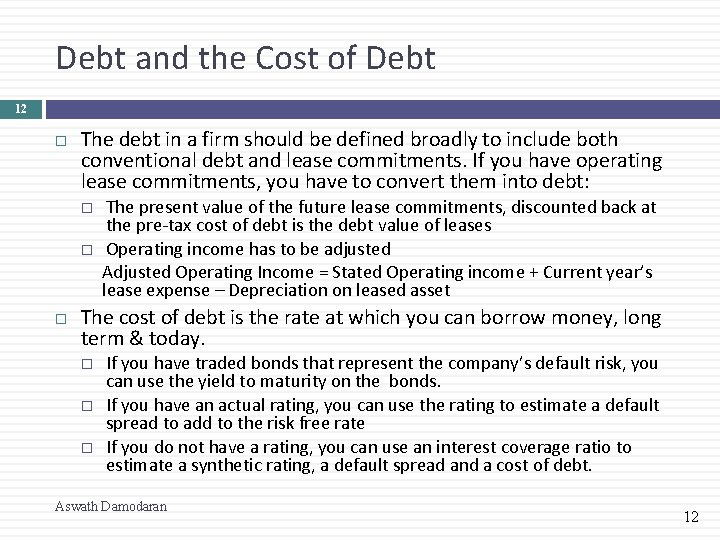Debt and the Cost of Debt 12 The debt in a firm should be defined broadly to include both conventional debt and lease commitments. If you have operating lease commitments, you have to convert them into debt: The present value of the future lease commitments, discounted back at the pre-tax cost of debt is the debt value of leases Operating income has to be adjusted Adjusted Operating Income = Stated Operating income + Current year’s lease expense – Depreciation on leased asset The cost of debt is the rate at which you can borrow money, long term & today. If you have traded bonds that represent the company’s default risk, you can use the yield to maturity on the bonds. If you have an actual rating, you can use the rating to estimate a default spread to add to the risk free rate If you do not have a rating, you can use an interest coverage ratio to estimate a synthetic rating, a default spread and a cost of debt. Aswath Damodaran 12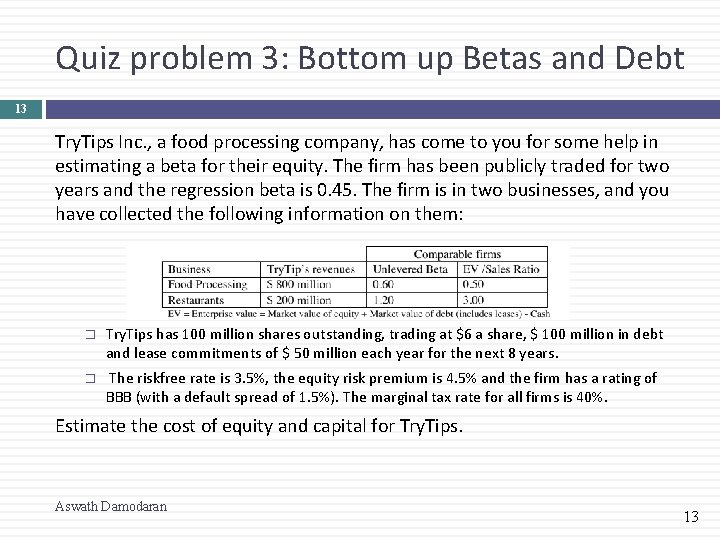Quiz problem 3: Bottom up Betas and Debt 13 Try. Tips Inc. , a food processing company, has come to you for some help in estimating a beta for their equity. The firm has been publicly traded for two years and the regression beta is 0. 45. The firm is in two businesses, and you have collected the following information on them: � Try. Tips has 100 million shares outstanding, trading at \$6 a share, \$ 100 million in debt and lease commitments of \$ 50 million each year for the next 8 years. � The riskfree rate is 3. 5%, the equity risk premium is 4. 5% and the firm has a rating of BBB (with a default spread of 1. 5%). The marginal tax rate for all firms is 40%. Estimate the cost of equity and capital for Try. Tips. Aswath Damodaran 13Solution 14 Estimate values of the two businesses � � � Next, estimate the value of debt � � � Value of food processing = 800*. 5 = 400 Value of restaurant = 200*3 = 600 Unlevered beta = 0. 60 (400/1000) + 1. 20 (600/1000) = 0. 96 Pre-tax cost of debt = 3. 5% +1. 5% =5% PV of leases = PV of \$50 million for 8 years @5% = \$323 m Total debt = \$100 + \$323 = \$423 m Finally, estimate the levered beta � � D/E ratio = 423/ 600 = 70. 53% Levered beta= 0. 96 (1+ (1 -. 4) (. 7053)) = 1. 366234212 Cost of equity = 3. 5% + 1. 366 (4. 5%) = 9. 65% Cost of capital = (600/1023) (9. 65%) + (423/1023) (5%(1 -. 4)) = 6. 90% Aswath Damodaran 14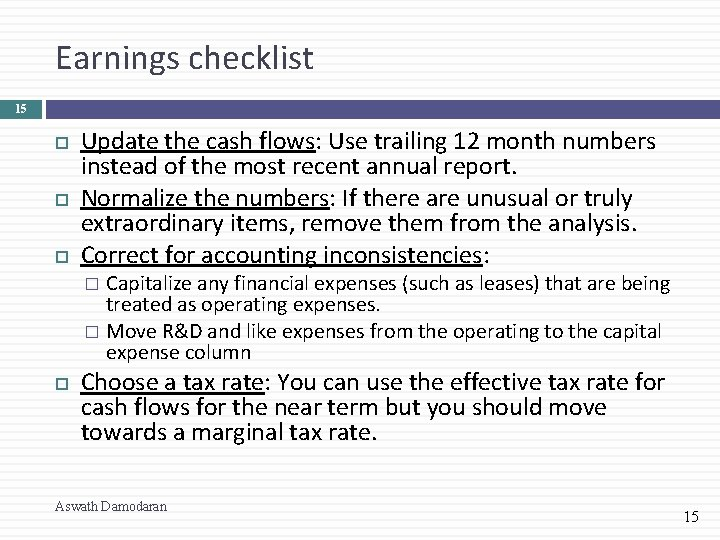Earnings checklist 15 Update the cash flows: Use trailing 12 month numbers instead of the most recent annual report. Normalize the numbers: If there are unusual or truly extraordinary items, remove them from the analysis. Correct for accounting inconsistencies: Capitalize any financial expenses (such as leases) that are being treated as operating expenses. � Move R&D and like expenses from the operating to the capital expense column � Choose a tax rate: You can use the effective tax rate for cash flows for the near term but you should move towards a marginal tax rate. Aswath Damodaran 15R&D Expenses: Operating or Capital Expenses 16 Accounting standards require us to consider R&D as an operating expense even though it is designed to generate future growth. It is more logical to treat it as capital expenditures. To capitalize R&D, Specify an amortizable life for R&D (2 - 10 years) � Collect past R&D expenses for as long as the amortizable life � Sum up the unamortized R&D over the period. (Thus, if the amortizable life is 5 years, the research asset can be obtained by adding up 1/5 th of the R&D expense from five years ago, 2/5 th of the R&D expense from four years ago. . . : � Aswath Damodaran 16Cash flow checklist 17 Net Capital Expenditures: Define these broadly to include not only the stated cap ex in the statement of cash flows but R&D expenses and acquisition costs. Working capital: Count only non-cash working capital investments. You may have to normalize this number, if it is volatile. Aswath Damodaran 17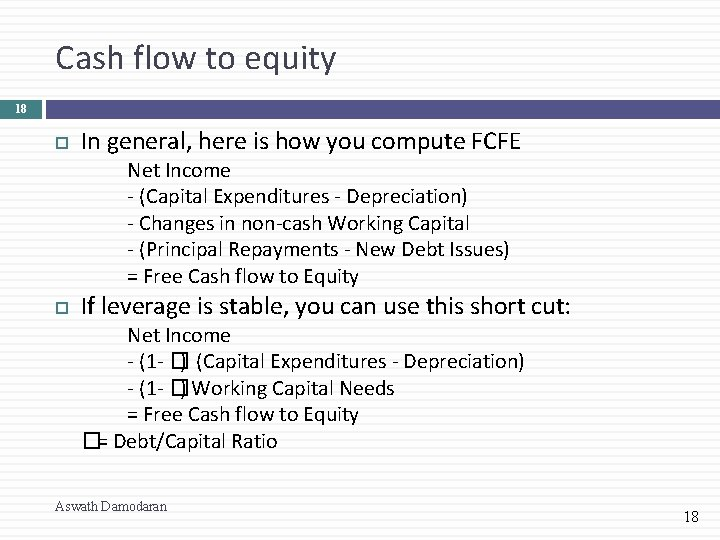Cash flow to equity 18 In general, here is how you compute FCFE Net Income - (Capital Expenditures - Depreciation) - Changes in non-cash Working Capital - (Principal Repayments - New Debt Issues) = Free Cash flow to Equity If leverage is stable, you can use this short cut: Net Income - (1 - �) (Capital Expenditures - Depreciation) - (1 - �) Working Capital Needs = Free Cash flow to Equity �= Debt/Capital Ratio Aswath Damodaran 18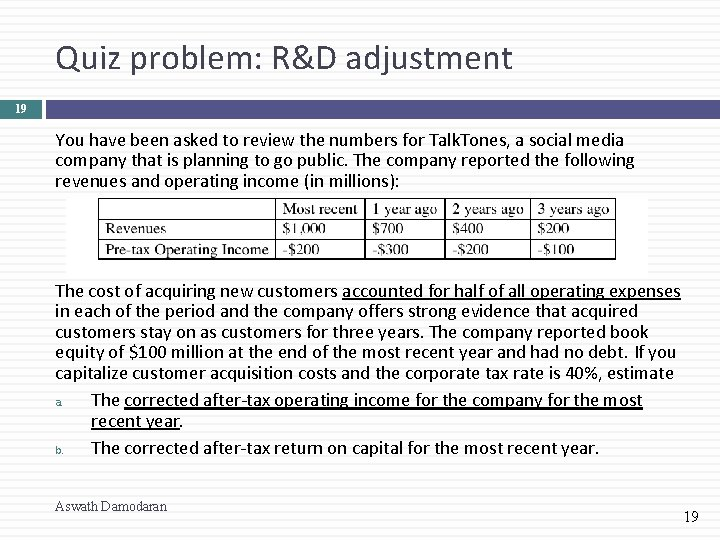Quiz problem: R&D adjustment 19 You have been asked to review the numbers for Talk. Tones, a social media company that is planning to go public. The company reported the following revenues and operating income (in millions): The cost of acquiring new customers accounted for half of all operating expenses in each of the period and the company offers strong evidence that acquired customers stay on as customers for three years. The company reported book equity of \$100 million at the end of the most recent year and had no debt. If you capitalize customer acquisition costs and the corporate tax rate is 40%, estimate a. The corrected after-tax operating income for the company for the most recent year. b. The corrected after-tax return on capital for the most recent year. Aswath Damodaran 19Solution 20 Corrected Book value of equity (capital) = 100 + 1000 = 1100 Corrected after-tax return on capital = 100/1100 = 9. 09% Aswath Damodaran 20